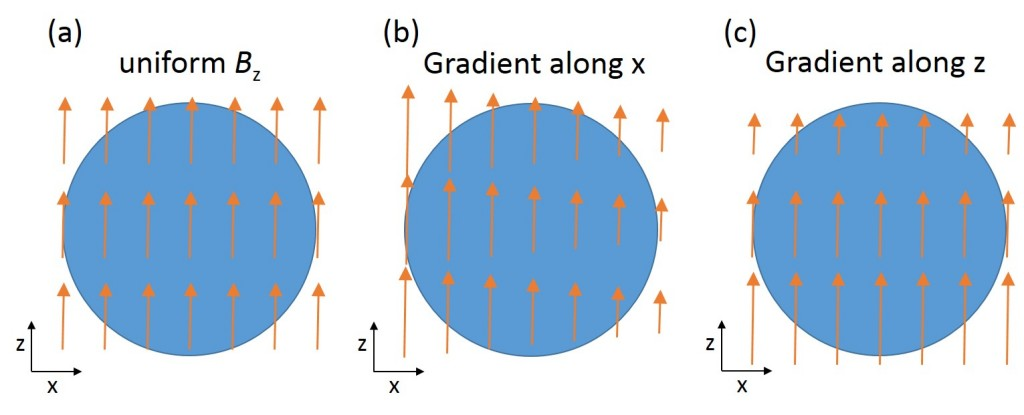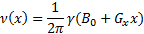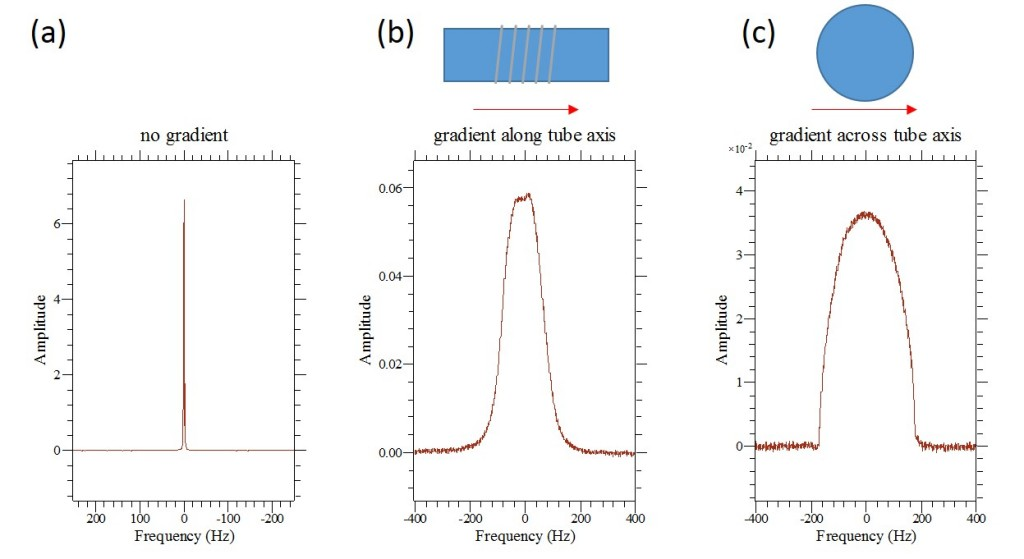# Gradients in NMR Spectroscopy – Part 1: The Basics

For high resolution NMR spectroscopy we aim to get the magnetic field as uniform as possible across the measurement volume. This manifests itself in narrow peaks in the spectrum and better spectral resolution. However, there are situations where field variations over the measurement volume (called Magnetic Field Gradients or MFG) can be beneficial. This blog post is the first part of a series of posts where we will describe the use of MFGs in the Spinsolve benchtop NMR spectrometer.

We normally change the magnetic field over the sample volume so that the strength of the field varies linearly along one direction. The effect of the MFG on the signal depends on the direction along which the field strength changes, so it is important to specify this. To illustrate this, Figure 1 (a) shows in blue a schematic of a transverse cut through the sample tube. The direction and strength of the magnetic field is indicated by the direction and length of the arrows. Note that for a uniform field the arrows have everywhere the same length and direction.Figure 1: Schematic diagram of a sample tube (blue) in a uniform magnetic field (a), and the same tube exposed to a magnetic field gradient (b, c).

Figure 1 (b) and (c) show examples, where the field strength (the length of the arrows) decreases with increasing distance x (orthogonal to the field direction) or z (along the field direction). A third case (which is not shown here) is where the variation is into the plane (y direction). Since the direction along which the field strength is important, we need to specify this direction. For example, if the variation is along the z direction, we call it a z gradient, or Gz, and similarly with x and y.

So what are the results showing us? If you look again at Figure 1 (b) and (c), you will see that different parts of the sample are exposed to a different magnetic field strength. Since the NMR frequency is directly proportional to the magnetic field strength, we get a direct mapping between spatial coordinate x and frequency ν:This means that if we acquire a spectrum of a sample exposed to a magnetic field gradient, the spectrum represents a one-dimensional image (or profile) of the sample! Figure 2 shows the measured spectra of a water sample in a uniform field (a), with a gradient along the tube axis (b), and a gradient transverse to the tube axis (c).Figure 2: (a) Measured spectrum of a water sample acquired in a uniform field. (b) Spectrum of the same sample acquired with a gradient along the tube axis. The characteristic hat shape reflects the excitation profile of the rf coil. (c) Spectrum of the same sample acquired with a gradient transverse to the tube axis. The shape reflects the circular profile of the sample.

This is the basis for how MRI scanners in hospitals work.

To conclude this post, let us summarise the important findings:

• A gradient is the linear variation of the magnetic field strength along a certain direction.
• Under a magnetic field gradient, the NMR frequency depends on the position.
• If we measure the spectrum of a sample under a gradient, the spectrum is a one-dimensional image, or profile, of the sample. This is how the NMR MOUSE, one of Magritek’s products, works.

Please keep your eyes open for the next post in this series which will deal with pulsed field gradients.